# How to Use the Fibonacci Trading Strategy to Consistently Make Money?

If you’re just getting started with trading, you’ve most probably heard of the famous Fibonacci retracement levels, such as the 61.8% level. However, there is more to trading Fibonaccis than simply opening trade as soon as the price reaches one of those levels.

Here is how to use Fibonaccis to create a consistent trading strategy in the markets.

## What is Fibonacci?

Leonardo Fibonacci was a famous Italian medieval mathematician who coined what is today known as the Fibonacci sequence of numbers. The sequence first appeared in the book Liber Abaci and is formed such that each number is the sum of the two preceding numbers, starting from 0 and 1.

This means, the Fibonacci sequence goes like this: 0, 1, 1, 2, 3, 5, 8, 13, 21, 34, 55, 89, 144…

The sequence, and various ratios based on the sequence, can be found in almost all corners of nature, from the proportions of the human body to seashells and the arrangement of leaves in sunflowers. In this regard, the 0.618 ratio, which is derived by dividing each number by its succeeding numbers (e.g. 89/144), is especially important and can be observed in many natural phenomena.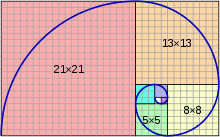Initially, Fibonacci used the sequence to describe the exponential growth of a rabbit population, but the sequence and ratios have also been used in many other disciplines since then, including trading.

## Are Fibonacci Levels Useful in Trading?

The Fibonacci sequence and ratios are extremely popular in trading the financial markets. Although there are still discussions among traders about why Fibonaccis work in trading, the truth is that there are many successful traders who rely on Fibonacci ratios in their daily trading.

In trading, Fibonacci levels are based on ratios that are derived from the Fibonacci sequence itself. The 61.8% level is arguably the most important Fibonacci level, but there are also other ratios such as the 23.6%, the 38.2%, the 50%, and the 78.6% levels.

The Fibonacci levels allow traders to visualize where an impulsive move of a trend may complete a pullback, before continuing in its original direction. In this regard, the Fibonacci tools have many touching points with the Dow theory, which states that a trend usually retraces around the 50% of its move before resuming its original direction.

Here is how the Fibonacci levels look like on a chart.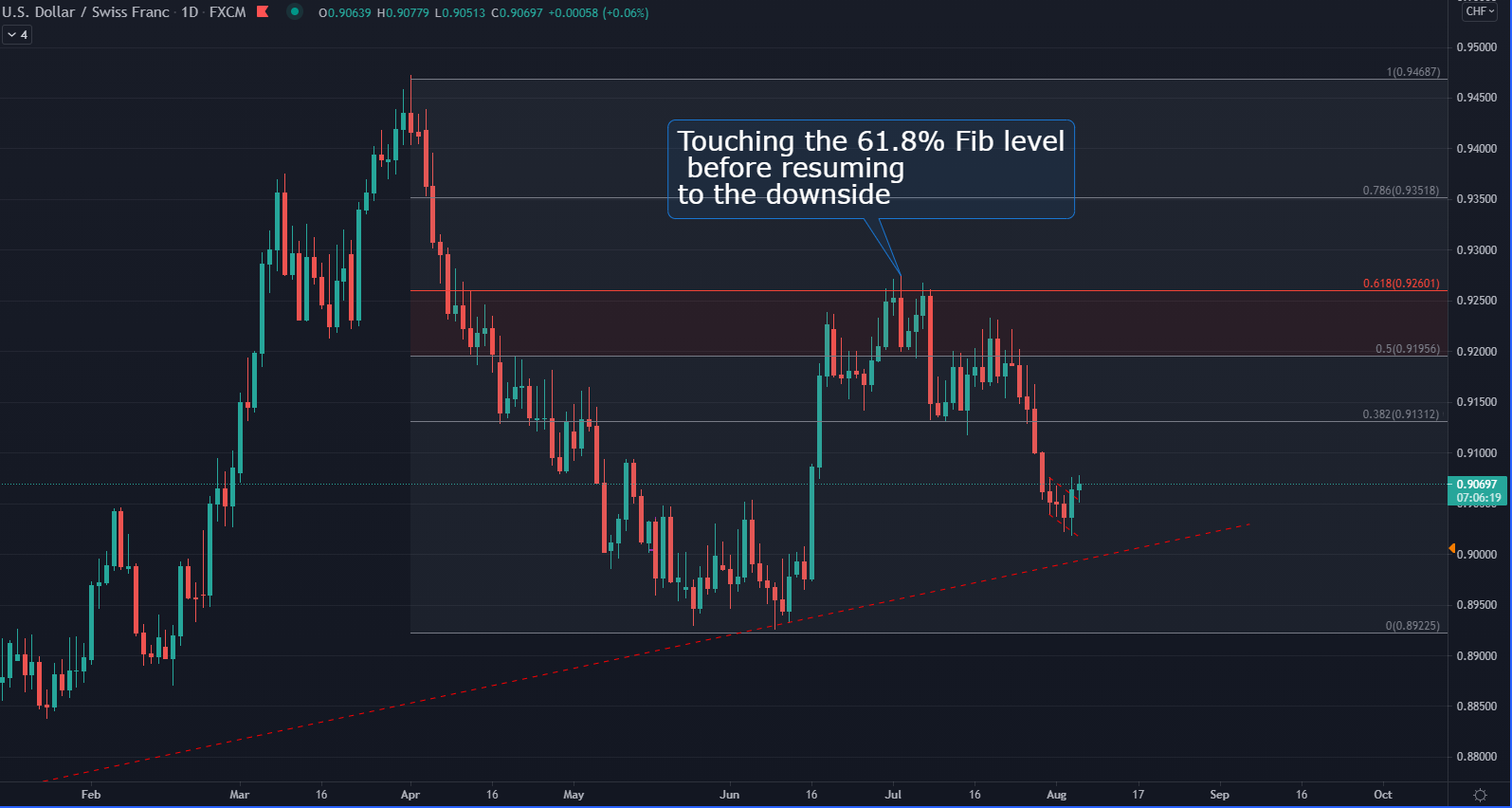When trading with Fibonacci levels, the key is to identify a trending market. Since Fibonacci levels are used to identify possible levels where trend corrections might end, the market has to be trending for Fibonacci levels to work. If you apply the levels to a ranging market, you’ll face many fake signals and possibly high losses if you follow the strategy in that market condition.

So far, you’ve learned that Fibonacci levels identify areas of high-probability reversals. As a rule of thumb, the stronger the underlying trend, the shallower the pullback.

This means that during strong trends, the price will trend to retrace off the lower Fibonacci levels, such as the 23.6% and 38.2% levels. Weaker trends will have deeper pullbacks and the price could reach the 50% Fib level, the 61.8% Fib level, or even the 78.6% Fib level.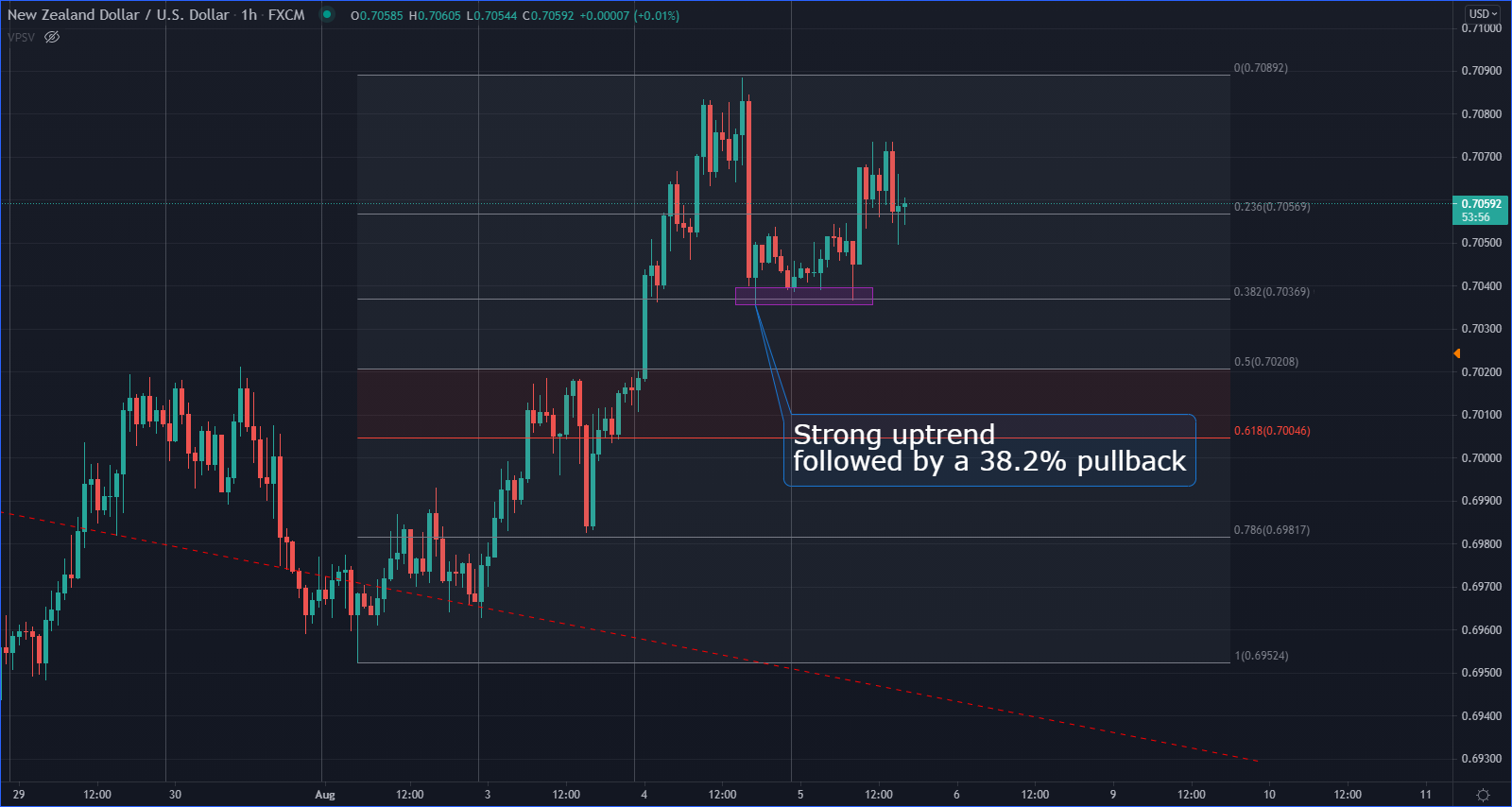The chart above shows the hourly chart of NZDUSD after the release of very strong labor market numbers in New Zealand. The pair rallied and completed a pullback at the 38.2% Fib, signaling a good buying opportunity and a strong bullish trend.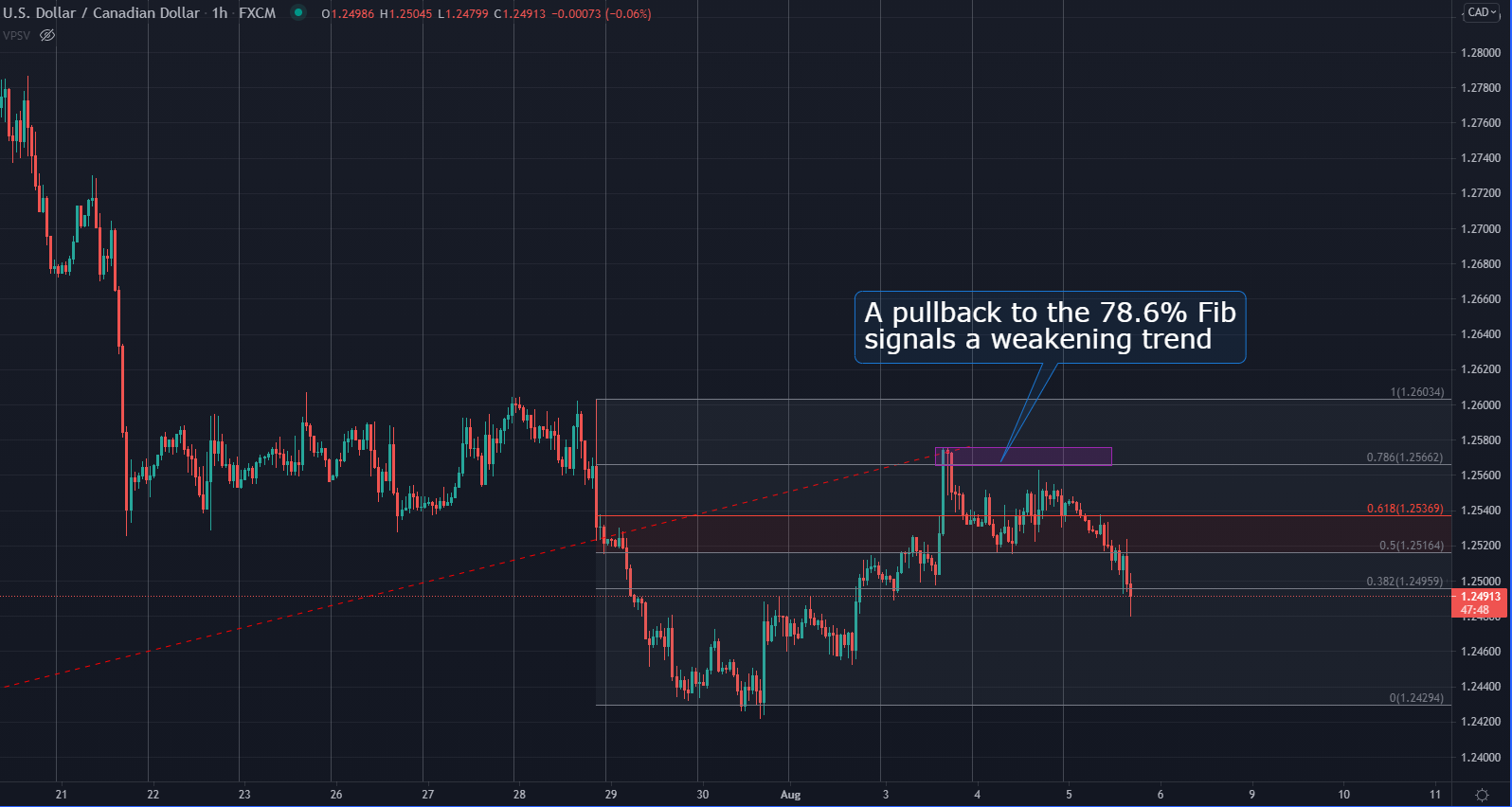The next chart shows the USDCAD pair which traded in a downtrend recently. However, the downtrend is apparently losing steam as the price completed a pullback all the way up to the 78.6% Fib level. Traders who follow Fibonacci levels should think about closing their selling position in USDCAD and preparing for a possible trend reversal to the upside.

## The Golden Zone

The middle of the Fibonacci range is often called the “golden zone” of retracements as many pullbacks reverse exactly inside the zone. The zone refers to the area between the 50% Fib level and the 61.8% Fib level, which is marked with red on the charts above.

Why do most reversals happen inside the golden zone? First, the golden zone lies in the middle of the Fibonacci levels, and according to the Dow theory, most trends retrace 50% of their impulse moves before continuing in their original direction.

Second, the 61.8% Fib level is arguably the most important Fib level of all, as it is calculated by simply taking one number from the Fibonacci sequence and dividing it by the succeeding number in the sequence. This makes the golden zone so important in trading.

Even though the golden zone offers great trading opportunities when trading with the Fibonacci tool, it’s very important to confirm a trade setup before opening a trade. Confirming a trade can be done with various technical tools, including candlestick patterns, chart patterns, and indicators. Since we focus mostly on price-action, here is an example of trading the golden zone with a chart pattern.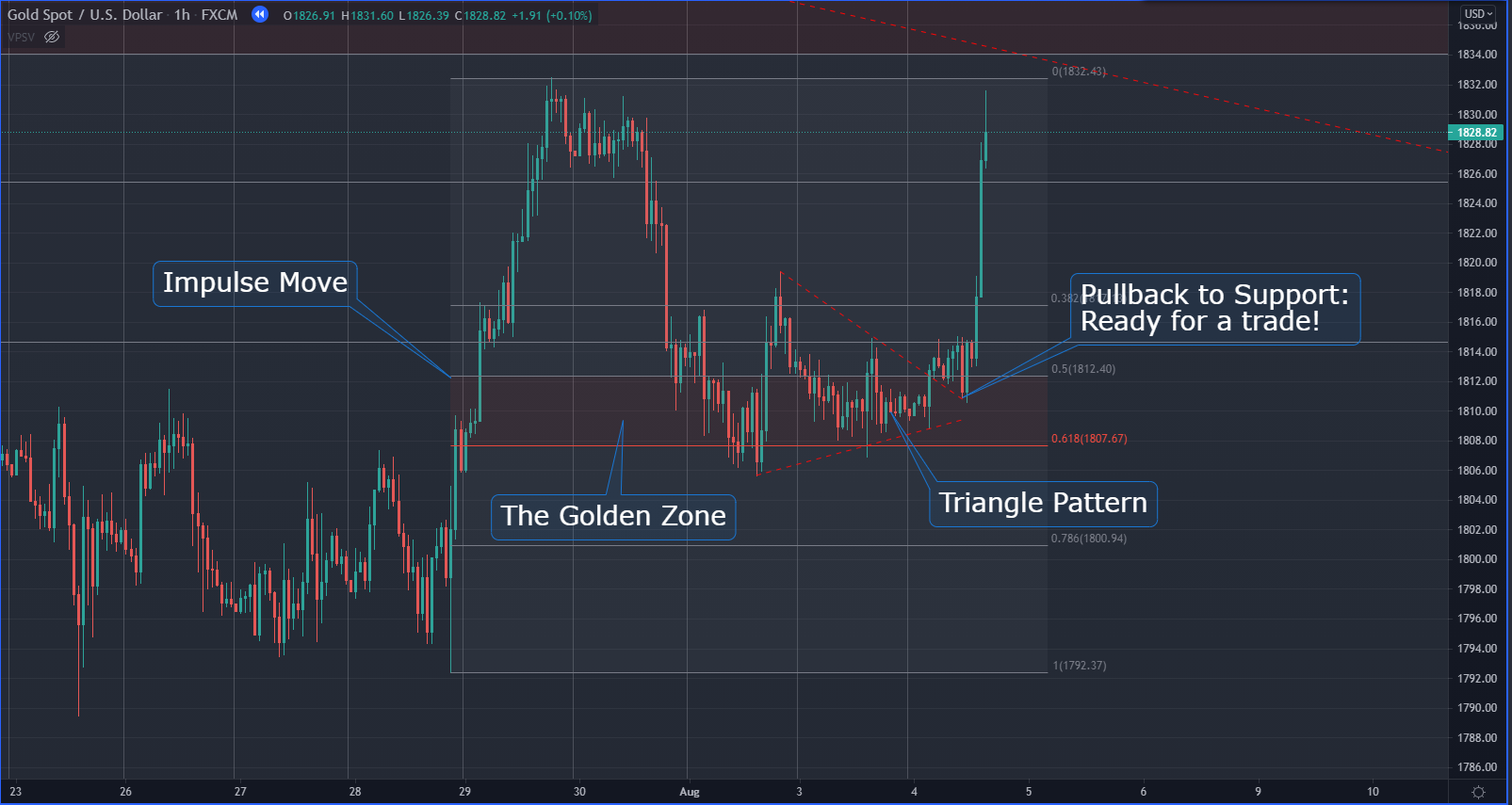As you can see from the hourly gold chart, the impulse move faced support near the 61.8% Fib level and traded sideways inside the golden zone. The sideways-moving market formed a triangle pattern that got broken the next day, followed by a pullback to the triangle’s upper trendline which then acted as support. A great trade based on the 50-61.8% zone and a triangle confirmation!

As usually when trading triangles, you could place a stop-loss just below the triangle, and aim for a profit target that is equal to the height of the triangle base. However, since we are trading trend continuations with Fibonaccis, it’s good to have a slightly higher profit target as well.

## Final Words

The Fibonacci tool is a great way to take advantage of trend-following strategies in the markets. The Fibonacci tool is based on the Fibonacci sequence of numbers and their ratios, most notably the 38.2% Fib level, the 61.8% Fib level, and the 78.6% Fib level.

While those levels offer a high chance of a possible reversal, you should always use other technical tools to confirm a trade setup before opening a position.